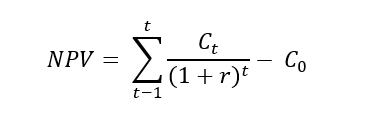# The Internal Rate of Return: Assessing the Profitability of Potential Investments# The Internal Rate of Return: Assessing the Profitability of Potential Investments

Long-term project investments differ from their short-term sisters in many ways. Risk, financing structure, and investment ticket size are just some of the aspects which need to be addressed according to a project’s lifetime. Furthermore, the profits of a long-term investment usually precede the respective fund transferal by a significant period of time, impacting the profitability of the potential investment.

Several approaches are in common practice among professionals to evaluate if the profits of an investment, and to assess if the potential profits justify the risks inherent in long-term investment. Two of the methods used in common practice are the Net Present Value (NPV) and the Internal Rate of Return (IRR).

The internal rate of return (IRR) can be defined as the rate of return that makes the net present value (NPV) of all cash flows equal to zero.
Calculation of the internal rate of return considering only the project cash flows results in the project IRR. The internal rate of return calculations relies on the same formula as the NPV and is represented by the following formula:Ct………………..net cash inflow during period t
C0………………. total initial investment costs
r………………….discount rate
t………………….number of time periods

To calculate IRR using this formula, the NPV would be set equal to zero and then one would solve for the discount rate r, giving the internal rate of return.

The IRR offers potential investors a means to assess the expected rate of return of the project and determine if the project will generate a positive margin for the shareholder. If a project has an IRR greater than its cost of capital would increasing its value to the shareholder.
Generally speaking, you can think of IRR as the rate of growth a project is expected to generate. Although the actual rate of return that the relevant project may ending up generating will often differ from its estimated IRR, a project with a substantially higher IRR than other available options would still provide a better chance of strong growth.

The IRR is a very popular metric used to estimate a project’s profitability. But as many key performance indicators, it can be misleading if used alone. Depending on the initial investment costs, a project may have a low IRR but a high NPV. Which can be the result of a slow moving project, where the company sees returns on that project in the rather distant future, but the relevant project may be adding a great amount of overall value to the company.
But generally speaking, investment decision based on NPV and IRR usually go hand in hand. If an investment proposal is feasible according to the NVP evaluation, it is very likely to be so, according to the IRR evaluation as well.

An issue concerning the IRR not strictly inherent to the metric itself, but rather to a common misuse of IRR, is the assumption that when positive cash flows are generated during the course of a project (not at the end), the money will be reinvested at the project’s rate of return. This rarely is the case. When and if positive cash flows are reinvested, it will be at a rate that more resembles the cost of capital. Miscalculating the IRR in this manner may lead to the assumption that a project is more profitable than it actually is.

Closing with the most crucial point in calculating an IRR, which is to estimate a projects cash flow, taking into account the difference between investment expenditures and yearly cash inflow when the project is operating.